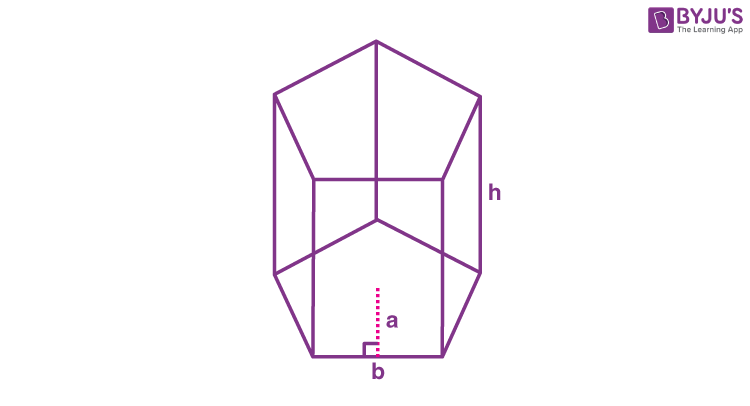# Pentagonal Prism

A pentagonal prism is a prism that has two pentagonal bases like top and bottom and five rectangular sides. It is a type of heptahedron with 7 faces, 10 vertices and 15 edges. A pentagonal prism can have pentagonal bases which give five sides. A pentagonal prism is also known as a five-sided polygon prism.

To recall, the prism shape, in maths, is a three-dimensional box i.e. a solid figure with a uniform cross-section and has two common bases.

• Face: A flat side of a 3-dimensional object
• Base: One of the two parallel and congruent sides of an object
• Edge: Intersection of two faces on a solid object. This is a line.
• Vertex: Joining point of two edge sides.

## Types of Pentagonal Prisms

There are two types of pentagonal prisms which are:

• Regular Pentagonal Prisms
• Right Pentagonal Prisms

### Regular Pentagonal Prism

If all the sides of the pentagonal prism are of equal length, then it is said to be a regular pentagonal prism. In a regular pentagonal prism, all the rectangular faces are congruent. The rectangular faces are said to be lateral when the pentagonal faces of the regular pentagonal prism are bases. The lateral side faces are called lateral edges.

### Rectangular  Pentagonal Prism

A prism is a right pentagonal prism when it has two congruent and parallel pentagonal faces and five rectangular faces that are perpendicular to the triangular ones.

## Volume and Surface Area of a Pentagonal Prime

The two important measures made on a pentagonal prism are to find its volume and surface area.### Volume of Pentagonal Prism Formula

To find the volume of a regular pentagonal prism, first, you have to find the apothem length (a). The apothem length is a measure from the centre of a polygon to the midpoint of any side. The formula to find the volume of a pentagonal prism is given as:

 Volume of pentagonal prism= (5/2)×a×b×h cubic units

Where,

• a = Apothem length of the pentagonal prism
• b = Base length of the pentagonal prism
• h = Height of the pentagonal prism

### Surface Area of Pentagonal Prism Formula

The surface area is the area that describes the material that will be used to cover a geometric solid shape. The formula to find the surface area of the pentagonal prism is given by:

 Surface area of pentagonal prism = 5ab + 5bh square units

Where,

• a = apothem length of the pentagonal prism
• b = base length of the pentagonal prism
• h = height of the pentagonal prism

### Example Question Based on Pentagonal Prism Formulas

Question: Find the surface area and volume of the pentagonal prism with apothem length of 6 cm, base length of 10 cm and height of 11 cm?

Solution:

Given,

Apothem Length of the pentagonal prism, a = 6 cm

Base length of the pentagonal prism, b = 10 cm

Height of the pentagonal prism, h = 11 cm

Volume and the surface area of a pentagonal prism

Volume of the pentagonal prism = (5/2)abh cubic units

= 5/2 × (6×10×11)

= 5/2 × (660)

= 5 × 330

= 1650

Therefore, the volume of the pentagonal prism is 1650 cm3

Surface area of pentagonal prism = 5ab + 5bh square units

= 5 (6×10) + 5 (10×11)

= 5(60) + 5(110)

= 300 + 550

= 850

Therefore, the surface area of a pentagonal prism is 850 cm2.

Q1

### What is Prism?

A prism can be defined as a three-dimensional shape that consists of two parallel, congruent faces called bases.

Q2

### What is a Pentagonal Prism?

A pentagonal prism can be defined as a prism having two pentagonal bases and five rectangular sides.

Q3

### What is the Formula for Surface Area of a Pentagonal Prism?

The surface area of a pentagonal can be found using the following formula:

A = 5ab + 5bh

Q4

### What is the Formula for the Volume of a Pentagonal Prism?

The volume of a pentagonal prism can be calculated using the following formula:

V = (5/2)×a×b×h

Test your Knowledge on Pentagonal Prism